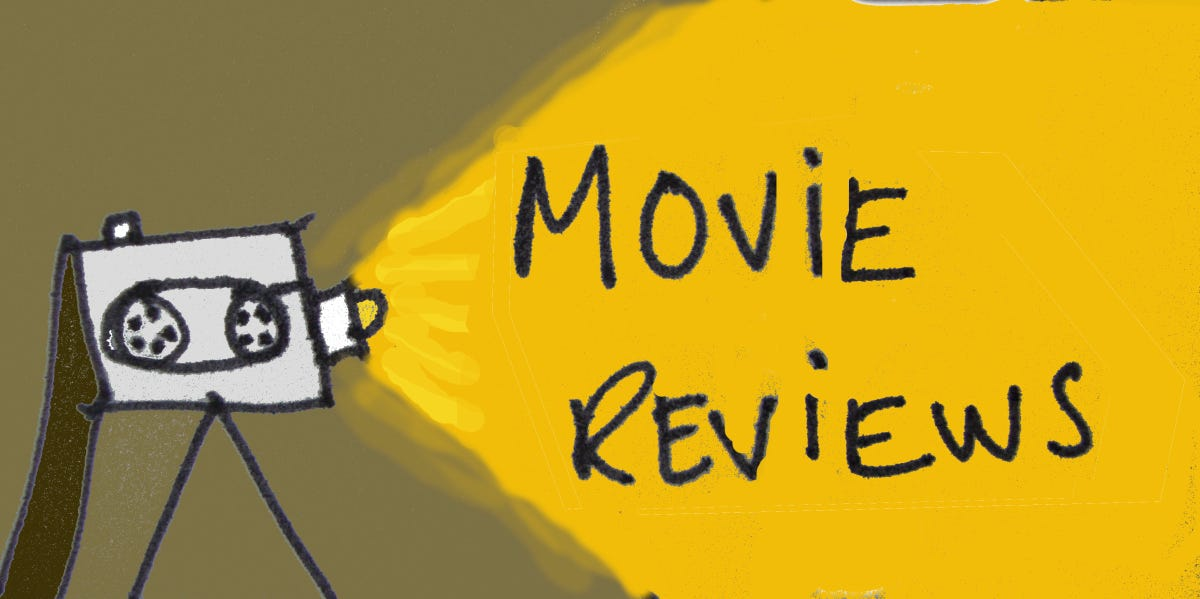# Text Classification with IMDb Movie Reviews# Text Classification with IMDb Movie Reviews

This Blog classifies movie reviews as positive or negative using the text of the review. This is an example of binary — or two-class — classification, an important and widely applicable kind of machine learning problem.

We’ll use the IMDB dataset that contains the text of 50,000 movie reviews from the Internet Movie Database. These are split into 25,000 reviews for training and 25,000 reviews for testing. The training and testing sets are balanced, meaning they contain an equal number of positive and negative reviews.

Import Libraries

The IMDB dataset is available Here.

# Explore the data

Let’s take a moment to understand the format of the data. Each example is a sentence representing the movie review and a corresponding label.

# Build the model

Let’s first create a Keras layer that uses a TensorFlow Hub model to embed the sentences

Let’s now build the full model:

# Loss function and optimizer

A model needs a loss function and an optimizer for training. Since this is a binary classification problem and the model outputs a probability (a single-unit layer with a sigmoid activation), we’ll use the `binary_crossentropy` loss function.

# Train the model

Train the model for 40 epochs in mini-batches of 512 samples. This is 40 iterations over all samples in the `x_train` and `y_train` tensors.

# Evaluate the model

This fairly naive approach achieves an accuracy of about 85%. With more advanced approaches, the model should get closer to 95%.

# Create a graph of accuracy and loss over time

In this plot, the dots represent the training loss and accuracy, and the solid lines are the validation loss and accuracy.

Notice the training loss decreases with each epoch and the training accuracy increases with each epoch. This is expected when using a gradient descent optimization — it should minimize the desired quantity on every iteration.